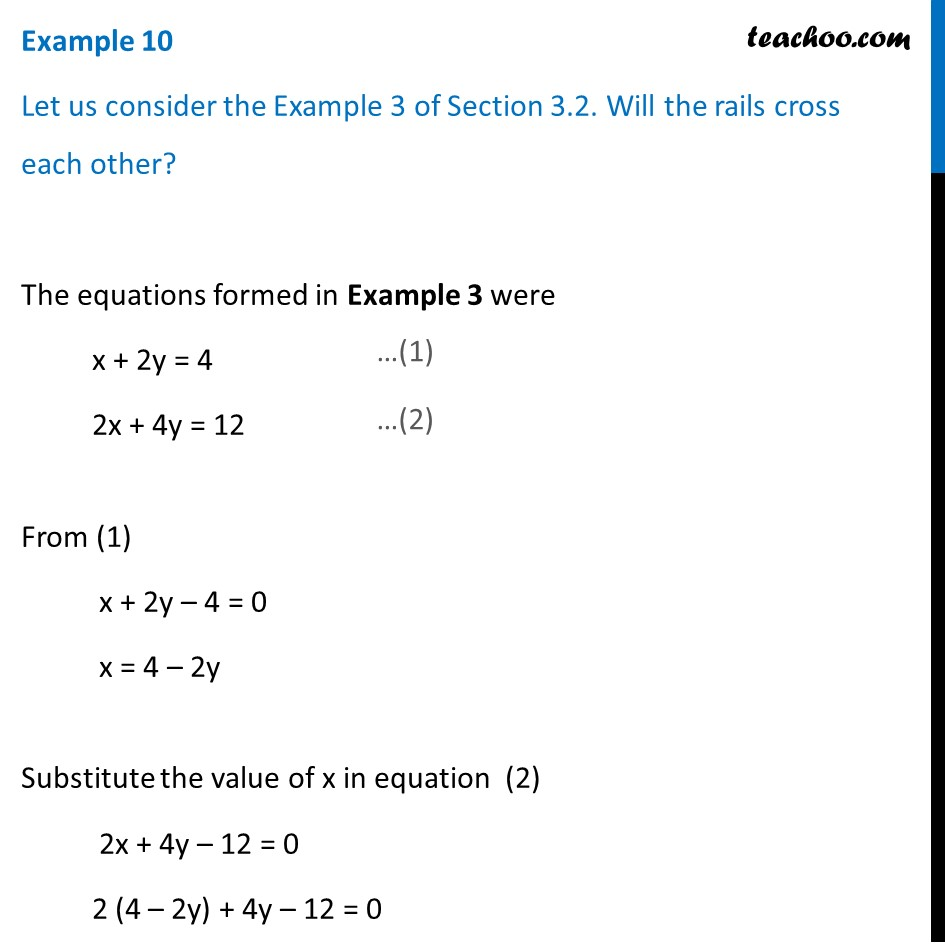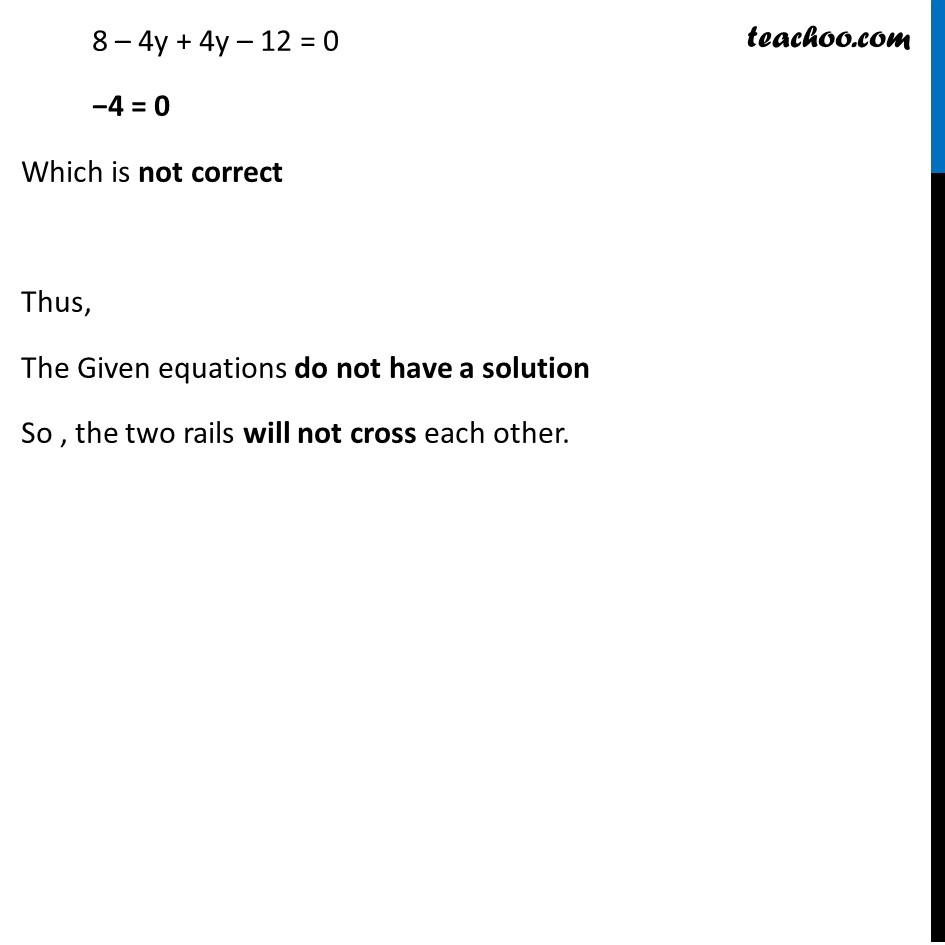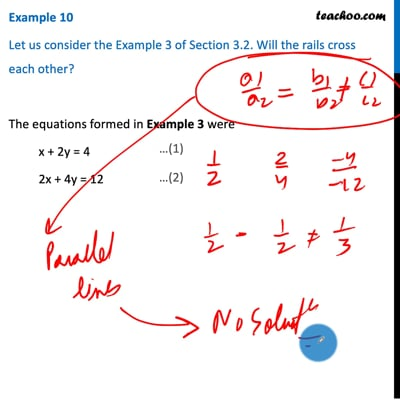Examples

Chapter 3 Class 10 Pair of Linear Equations in Two Variables
Serial order wiseThis video is only available for Teachoo black users

Solve all your doubts with Teachoo Black (new monthly pack available now!)

### Transcript

Example 10 Let us consider the Example 3 of Section 3.2. Will the rails cross each other? The equations formed in Example 3 were x + 2y = 4 2x + 4y = 12 From (1) x + 2y – 4 = 0 x = 4 – 2y Substitute the value of x in equation (2) 2x + 4y – 12 = 0 2 (4 – 2y) + 4y – 12 = 0 8 – 4y + 4y – 12 = 0 −4 = 0 Which is not correct Thus, The Given equations do not have a solution So , the two rails will not cross each other.